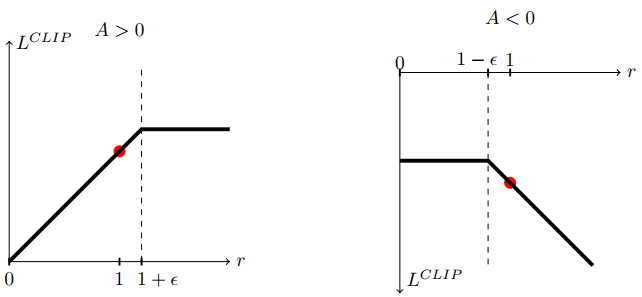## On-Policy vs. Off-Policy

• motivation
• 假设我们想要一个deterministic的policy，那么按一般的思路，我们就应该用一个deterministic的policy去采样，根据采样结果得到q(s, a)，最后得到$\pi (s) = \text{argmax}_a q(s,a)$。
• 但由于这样的采样过程是greedy的，容易采样不充分，陷入局部最优。
• 因此就想能不能既evaluate一个deterministic的policy，又可以充分采样，由此引出Off-Policy。
• idea（以sarsa和q-learning为例，阐述On-Policy与Off-Policy的区别）
• 无论是sarsa还是q-learning，我们真实采取的policy（i.e., 状态变换所依赖的behavior policy）都是对q(s, a)进行$\epsilon \text{-greedy}$。sarsa和q-learning的区别在于q(s, a)的含义不同。
• 假设t时刻到t+1时刻的state, action, reward如下表所示：
$$\begin{array}{c|cc} & t & t+1 \\ \hline R & & r’ \\\ S & s & s’ \\\ A & a & a’ \end{array}$$当我们要更新q(s, a)时，sarsa与q-learning有相同的$s, a, r’, s’$，但他们的$a’$并不相同，也正是$a’$的不同导致了不同的q(s, a)含义：
• sarsa通过对q($s’$, $A$)进行$\epsilon \text{-greedy}$得到$a’$，因此sarsa的q(s, a)表示$\epsilon \text{-greedy}$下的q-value；
• q-learning则通过$a’=\text{argmax}_A q(s’, A)$得到$a’$，因此q-learning的q(s, a)表示greedy（deterministic）下的q-value。
• 也就是说，在sarsa中，q(s, a)表示的是behavior policy的q-value；而q-learning中，q(s, a)表示的是另外一个policy的q-value。前者这种q(s, a)与behavior policy一致的就称为On-Policy，后者这种不一致的就称为Off-Policy。即划分On-Policy与Off-Policy的依据是q(s, a)对应的policy是不是behavior policy。更准确来说，假设更新$Q^{\pi}(s, a)$时，所用的样本$s, a \sim \pi ‘$，那么划分On-Policy与Off-Policy的依据是$\pi ‘$是否为$\pi$（因此experience replay基本都为Off-Policy，因为q(s, a)对应当前policy，而用到的sample来自experience replay，因此属于以前的policy）。
• more
• 上面说的是Off-Policy与On-Policy两者概念上的区分，至于q-learning这种做法能不能保证q(s, a)收敛，q(s, a)收敛的值是不是我们想要的值，则是另外一个问题了。

• motivation
• value based的方法难以处理continuous action space。因为即使得到了准确的q(s, a)，在给定一个状态s的情况下，仍然不知道该采取哪个action，因此一般的处理方法是discretization，
• 但是discretization在某些action space中并不合适（e.g., 表示旋转的四元数），
• 即使合适，discretization的程度也比较empirical。
• value based的方法难以处理随机策略（尽管可以根据q(s,a)来生成一个distribution（e.g., softmax distribution），但是并没有什么理论保证这种distribution是合理的）。
• 有些任务的policy比action value更容易approximate。
• idea
• 大多数情况下，我们的目的都是得到policy，value function只是得到policy的一种手段。
• 实际上我们可以直接对policy建模并求解最优policy。设policy为$\pi(a \mid s ; \boldsymbol\theta)$（e.g., 高斯分布$a \sim \mathcal{N}(\phi(s)^T \boldsymbol\theta, \sigma ^2)$），则最优policy（i.e., $\boldsymbol\theta$）为以下优化问题的解：
$$\boldsymbol\theta = \mathop{\text{argmax}}\limits_{\boldsymbol\theta} v_{\pi_{\boldsymbol\theta}}(s_0),$$此处我们假设每次的初始状态都为$s_0$。
• 假如知道$\nabla v_{\pi_{\boldsymbol\theta}}(s_0)$，那么我们就可以使用迭代的方法（e.g., SGD）去求解上述优化问题。
$$\nabla v_{\pi_{\boldsymbol\theta}}(s_0)=\mathbb{E}_{S_t, A_t \sim \pi}\left[\gamma ^t G_t \frac{\nabla _\boldsymbol\theta \pi (A_t \mid S_t, \boldsymbol\theta)}{\pi(A_t \mid S_t, \boldsymbol\theta)} \right],$$因此我们可以通过sample来估计$\nabla v_{\pi_{\boldsymbol\theta}}(s_0)$从而求解优化问题，得到最优policy。
• more
• 分析baseline对bias与variance的影响：
• 没有增加bias，且baseline越接近v(s)，variance越低；
• sample越independent，他的这种分析方法越准确。
• 插句题外话，原来不止我一个人觉得rl的公式推导很随意，期望/求导之类的顺序想换就换：）。

## Actor-Critic

• motivation
• Policy Gradient Theorem中的$G_t=q_{\pi}(S_t, A_t)$，也就是说在式子中$G_t$是真实的q(s,a)，这就限制了Policy Gradient只能用Monte Carlo的方法来采样。
• 而Monte Carlo方法的variance是比较高的（因为多步取样），甚至在某些情况下Monte Carlo方法并不适用（e.g., non-terminating problem）。
• 所以要想办法处理$G_t$。
• idea
• 一种直观的解决思路是求解一个近似的$G_t$，即找一个$v_{\boldsymbol w} \approx G_t$，然后用$v_{\boldsymbol w}$带入Policy Gradient中的$G_t$。
• 这个$v_{\boldsymbol w}$就是所谓的critic，而原来的policy就是所谓的actor。

## High-dimensional Continuous Control Using Generalized Advantage Estimation

• motivation
• GAE则给出了如何通过一个参数$\lambda$来调控这个tradeoff。
• idea
• $\lambda = 1$时：等价于用真实return-v(s)，不引入bias，但variance高。
• $\lambda = 0$时：假如v(s)不等于真实的v(s)，则会引入bias，但variance低。
• more
• discounted factor的另一种理解：即假设原来的目标是求undiscounted return，引入discounted factor是为了降低variance。

## Asynchronous Methods for Deep Reinforcement Learning

• motivation
• 消除sample之间的相关性的另一种方法（前一种是replay buffer）。
• 回顾我们的求解思路
1. 我们首先得到要优化的目标，通常是个期望值；
2. 然后基于采样得到的sample估计该目标值（依据是大数定理）；
3. 接着假装这个估计的值为真的目标，对它进行优化。
• 可以看到对目标值估计的准确与否直接决定了效果的好坏，而对目标值估计的准确与否取决于得到的sample是否满足大数定理的条件，即sample是否独立同分布（期望值对应的分布）。
• idea
• 不同actor并行地在各自的trajectory中采样。参数更新的框架跟单线程的是一样的：
• minibatch：用local的t来模拟（因为对minibatch的求导本来就是sum的形式，因此可以通过累加的形式来模拟）;
• target update：用global的T来模拟。

• motivation
• Policy Gradient通过直接求解最优的stochastic policy解决continuous action space的问题，但在某些需要deterministic policy的场景下并不适用（e.g., 机器人控制）。
• idea
• 仅看推导结果：
• determinisitc policy gradient的方向大致为$q(s, \pi (s))$增大的方向，即$\mathbb{E}_{s \sim \pi}\left[ \nabla _ \theta \pi(s) \nabla _a Q^{\pi}(s, a) \mid _{a=\pi(s)} \right] \approx \mathbb{E}_{s \sim \pi}\left[ \nabla _\theta Q^{\pi}(s, \pi(s)) \right]$（用约等于号是因为忽略了$\nabla _\theta Q^{\pi}(s,a)$这一项）；
• 而policy gradient的方向大致为$q(s, a)\text{log} \pi(s)$增大的方向，即$\mathbb{E}_{s,a \sim \pi}\left[ Q^{\pi}(s, a) \nabla _\theta \text{log} \pi(s) \right] \approx \mathbb{E}_{s,a \sim \pi}\left[ \nabla _\theta Q^{\pi}(s, a) \text{log} \pi (s) \right]$（同样忽略了$\nabla _\theta Q^{\pi}(s,a)$这一项）。
• more
• 相比Policy Gradient，Determinisitc Policy Gradient还有一个好处就是sampe efficiency更高，毕竟gradient中少了关于action的期望（i.e., 仅仅是$\mathbb{E}_{s}，而不是\mathbb{E}_{s,a}$）。但这也会使得exploration不足，所以一般又会采用Off-Policy的方法弥补这个缺陷。
• 而Off-Policy Actor-Critic的目标居然是$\mathbb{E}_{s \sim \beta}\left[ V^{\pi}(s) \right]$（而不是$\mathbb{E}_{s \sim \pi}\left[ V^{\pi}(s) \right]$），即state的分布由behavior policy决定。我个人认为这个是一个无奈之下的折中做法，因为最理想的情况是$\nabla _ \theta \mathbb{E}_{s \sim \pi}\left[ V^{\pi}(s) \right] = \mathbb{E}_{s, a \sim \beta} \left[ \sim \right]$（即可以用behavior policy采取的样本去估计真实的performance的gradient），但这样做的话，最后要显式给出不好求的state分布概率$\rho ^{\beta}(s)$，于是退而求其次，换了个好求的目标函数。

• motivation
• 在DPG的基础上引入deep learning，使critic和actor的表达能力更强
• idea（借鉴DQN）
• replay buffer：打破数据之间的相关性。值得注意的是，我们原来的目标是要最大化期望，而我们是通过采样来近似这个期望的。仅当我们的采样点是相互独立的，才能更好地近似期望值。于是使用一个replay buffer来把(s, a, r, s’)存起来，到更新参数的时候再从中抽取mini batch，这样就可以获得相关性较弱的数据。
• target network：保证ground truth的一致性。critic实际上是supervised learning，即用一个神经网络去做回归。如果相同的输入对应不同的输出，则无法收敛。于是就将生成ground truth所依赖的$Q(s, a)$和$\mu(s)$都做了一个备份，来保证相同的输入有相同的输出，从而保证critic能够收敛。得到critic后，actor就跟DPG一样，朝着使得critic增大的方向更新参数就好了。
• 这里有个地方比较confusing，因为在DPG中，critic的含义是在当前actor下的return估计（i.e., $V^{\pi}$）；而使用了target network后，critic的含义更像是在target actor下的return估计（i.e., $V^{\pi ‘}$）。那在这种情况下，actor还应该朝着critic增大的方向更新参数吗？我的理解是$\pi ‘$是逐步逼近$\pi$的，所以两者比较相似，差别不大。
• more
• DDPG和DPG在本质上的区别是换了一个能力更加强的critic。

## Trust Region Policy Optimization

• motivation
• idea
• 通过一系列的定理，找到了新旧policy对应的performance之差的下界
$$\eta(\pi_{\theta _{\text{new}}}) - \eta(\pi_{\theta _{\text{old}}}) \geq f(\theta _{\text{new}}).$$
• 通过最大化下界来间接提高$\eta(\pi_{\theta _{\text{new}}})$（这里有个疑惑就是怎么保证rhs>0的？）。
• detail
• 本来是一个无约束优化问题，但是求解该无约束优化问题得到的$\theta _{\text{new}} - \theta _{\text{old}}$很小（这应该是一个经验性的结果，但也很合理，因为目标函数里面有一项负的KL divergence，如果step size大了，该项会很小。但我有个疑问是step size小又有什么问题呢？）。于是把无约束优化问题转换成有约束优化问题。
• 最后得到的优化问题的目标函数和约束条件都是期望，需要通过采样来进行估计。
• 为了提高求解优化问题的效率，分别对目标函数和约束条件进行一阶近似和二阶近似，得到一个凸优化问题，因此是一个强对偶问题。于是可以通过求解对偶问题来求解原问题。
• 思路是求解对偶问题，但并不完全按照求解对偶问题的过程进行（我觉得是按照标准流程不好解，因为涉及到求解$x^T A x = b$）。
• 通过对$\theta _{\text{new}}$求导等于$0$得到$\theta _{\text{new}}-\theta _{\text{old}} = \frac{1}{\lambda}F^{-1}g$，其中$F$为KL divergence在$\theta _{\text{old}}$的Hessian matrix，$g$为目标函数在$\theta _{\text{old}}$的一阶导数，至此知道了$\theta _{\text{new}} - \theta _{\text{old}}$的方向。
• 通过对$\lambda$求导等于$0$，可得$(\theta _{\text{new}}-\theta _{\text{old}})^T F (\theta _{\text{new}}-\theta _{\text{old}})=\delta$，但这并不好求。回想上一步我们已经知道$\theta _{\text{new}} - \theta _{\text{old}}$的方向，于是直接在保证满足约束条件下做line search，这也就是scaling步所做的事情。
• 可以看到整个求解过程最核心的是求解$x=F^{-1}g$，又$F$是一个对称正定矩阵，可以用conjugate gradient来求解该线性方程。而conjugate gradient并不需要显式地使用$F$，仅需要$F$与某个向量$p$的乘积$Fp$。幸运的是，真的有一些方法能够在不使用$F$的情况下求解出$Fp$（仅需要求一阶导，而一阶导又有auto-differentiation工具可用）。所以我们能够在不用显式求出$F$的情况下求解$x=F^{-1}g$。
• references

## Proximal Policy Optimization Algorithms

• motivation
• 进化版的TRPO
• 实现更加简单；
• 适用于更多网络结构（parameter sharing between critic and actor）。
• idea
• TRPO约束条件的含义是在状态s下，新旧两个policy不要相差太远（以KL divergence为度量）。实际上就是担心为了提高$\frac{\pi _{\theta _\text{new}}(a \mid s )}{\pi _{\theta _\text{old}}(a \mid s )}\hat{A}(s,a)$而大幅度改变$\theta _{\text{new}}$。具体来说就是当$\hat{A}(s, a)>0$时，通过大幅提高$\pi _{\theta _\text{new}}(a \mid s)$来提高目标值；反之，当$\hat{A}(s, a)<0$时，通过减小$\pi _{\theta _\text{new}}(a \mid s)$来提高目标值。
• PPO就是通过取minimize以及clip的方法改变了目标值的函数图像，使得大的step size也无法提高目标值，从而间接限制了step size，进而将约束问题转化为无约束优化问题。• more
• 关于新的目标函数的求导：
• 写成根据A>0还是A<0分类讨论的形式；
• 直接用auto-differentiation工具。
• 处理parameter sharing：总的objective中增加value function的objective（i.e., value function的回归），变成多目标优化问题。
• entropy bonus：鼓励exploration。